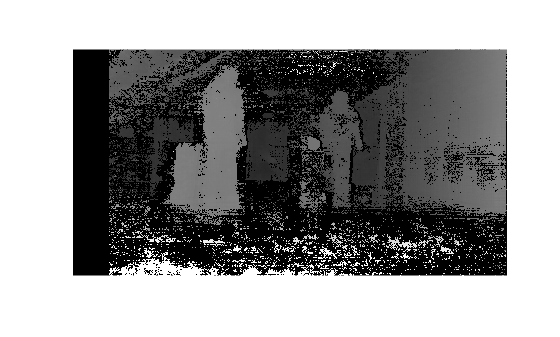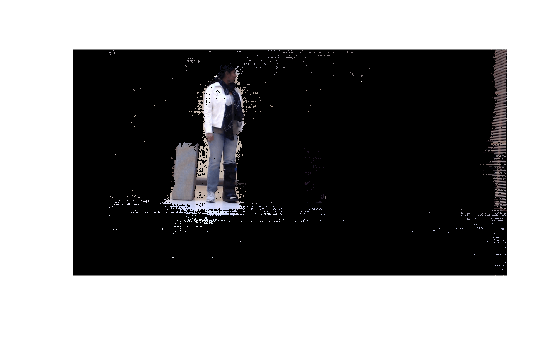# reconstructScene

Reconstruct 3-D scene from disparity map

## Syntax

``xyzPoints = reconstructScene(disparityMap,reprojectionMatrix)``

## Description

example

````xyzPoints = reconstructScene(disparityMap,reprojectionMatrix)` returns an array of 3-D world point coordinates that reconstruct a scene from a disparity map. The 3-D world coordinates are relative to the optical center of camera 1 in a stereo system. You can use the `rectifyStereoImages` function to obtain the `reprojectionMatrix` from a pair of stereo images.```

## Examples

collapse all

Load the stereo parameters.

`load('webcamsSceneReconstruction.mat');`

Read in the stereo pair of images.

```I1 = imread('sceneReconstructionLeft.jpg'); I2 = imread('sceneReconstructionRight.jpg');```

Rectify the images.

`[J1, J2, reprojectionMatrix] = rectifyStereoImages(I1,I2,stereoParams);`

Display the images after rectification.

```figure imshow(cat(3,J1(:,:,1),J2(:,:,2:3)),'InitialMagnification',50);```Compute the disparity.

```disparityMap = disparitySGM(im2gray(J1),im2gray(J2)); figure imshow(disparityMap,[0,64],'InitialMagnification',50);```Reconstruct the 3-D world coordinates of points corresponding to each pixel from the disparity map.

`xyzPoints = reconstructScene(disparityMap,reprojectionMatrix);`

Segment out a person located between 3.2 and 3.7 meters away from the camera.

```Z = xyzPoints(:,:,3); mask = repmat(Z > 3200 & Z < 3700,[1,1,3]); J1(~mask) = 0; imshow(J1,'InitialMagnification',50);```## Input Arguments

collapse all

Disparity image, specified as a 2-D array of disparity values for pixels in image 1 of a stereo pair. You can use either `disparityBM` or `disparitySGM` functions to generate the disparity image.

The disparity image can contain invalid values marked as `NaN`. These values correspond to pixels in image 1 that did not match with image 2. The function sets the world coordinates corresponding to invalid disparity value to `NaN`.

Pixels with zero disparity correspond to world points that are too far away to measure, given the resolution of the camera. The function sets the world coordinates corresponding to zero disparity to `Inf`.

When you specify the `disparityMap` input as a `double`, the function returns the coordinates as `double`. Otherwise, the function returns the coordinates as `single`.

Data Types: `single` | `double`

Reprojection matrix, specified as a 4-by-4 matrix. You can use the `rectifyStereoImages` function to obtain the `reprojectionMatrix` from a pair of stereo images.

The reprojection matrix is represented as:

`$\left[\begin{array}{cccc}1& 0& 0& -cx\\ 0& 1& 0& -cy\\ 0& 0& 0& f\\ 0& 0& 1/b& 0\end{array}\right]$`

where f and [cx,cy] are the focal length and principal point of the rectified camera 1, respectively. b is the baseline of the virtual rectified stereo camera.

Data Types: `single` | `double`

## Output Arguments

collapse all

Coordinates of world points, returned as an M-by-N-by-3 array. The 3-D world coordinates are relative to the optical center of camera 1 in the stereo system represented by `stereoParams`.

The output array contains the [x, y, z] coordinates of world points that correspond to the pixels in the `disparityMap` input. `xyzPoints`(:, :, 1) contains the x world coordinates of points corresponding to the pixels in the disparity map. `xyzPoints`(:, :, 2) contains the y world coordinates, and `xyzPoints`(:, :, 3) contains the z world coordinates. The 3-D world coordinates are relative to the optical center of camera 1 in the stereo system.

When you specify the `disparityMap` input as `double`, the function returns the `xyzPoints` output as `double`. Otherwise, the function returns it as `single`.

Data Types: `single` | `double`

 G. Bradski and A. Kaehler, Learning OpenCV : Computer Vision with the OpenCV Library, Sebastopol, CA: O'Reilly, 2008.